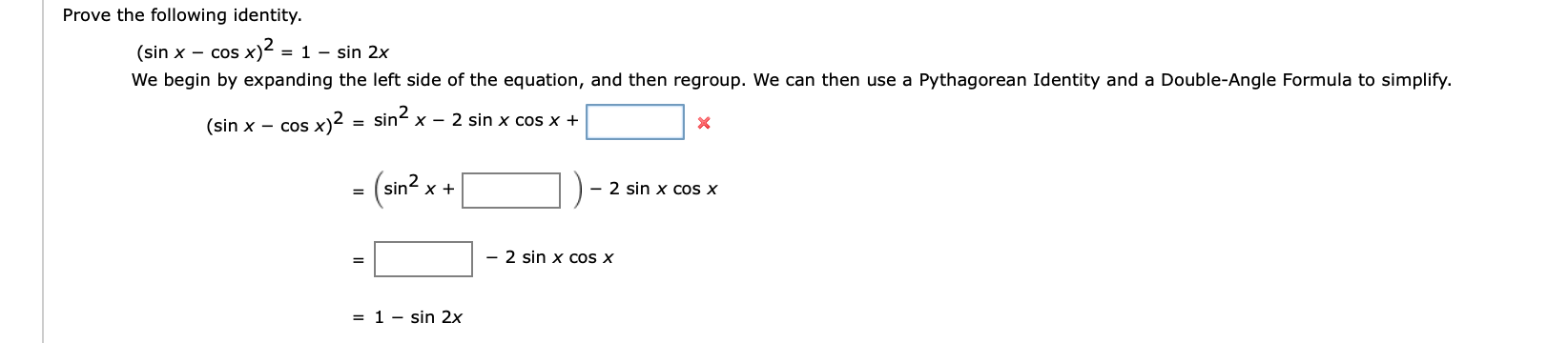# Prove the following identity.(sin x - cos x) = 1 - sin 2xWe begin by expanding the left side of the equation, and then regroup. We can then use a Pythagorean Identity and a Double-Angle Formula to simplify.sinx2 sin x cos x +(sin x cos x)2 :(sin2-2 sin x cos x=- 2 sin x COs X= 1 - sin 2x

Question
3 viewshelp_outlineImage TranscriptioncloseProve the following identity. (sin x - cos x) = 1 - sin 2x We begin by expanding the left side of the equation, and then regroup. We can then use a Pythagorean Identity and a Double-Angle Formula to simplify. sinx2 sin x cos x + (sin x cos x)2 : (sin2 -2 sin x cos x = - 2 sin x COs X = 1 - sin 2x fullscreen
check_circle

Step 1

Use (a-b)^2= a^2 -2ab+ b^2 formula to expand i...

### Want to see the full answer?

See Solution

#### Want to see this answer and more?

Solutions are written by subject experts who are available 24/7. Questions are typically answered within 1 hour.*

See Solution
*Response times may vary by subject and question.
Tagged in

### Trigonometry